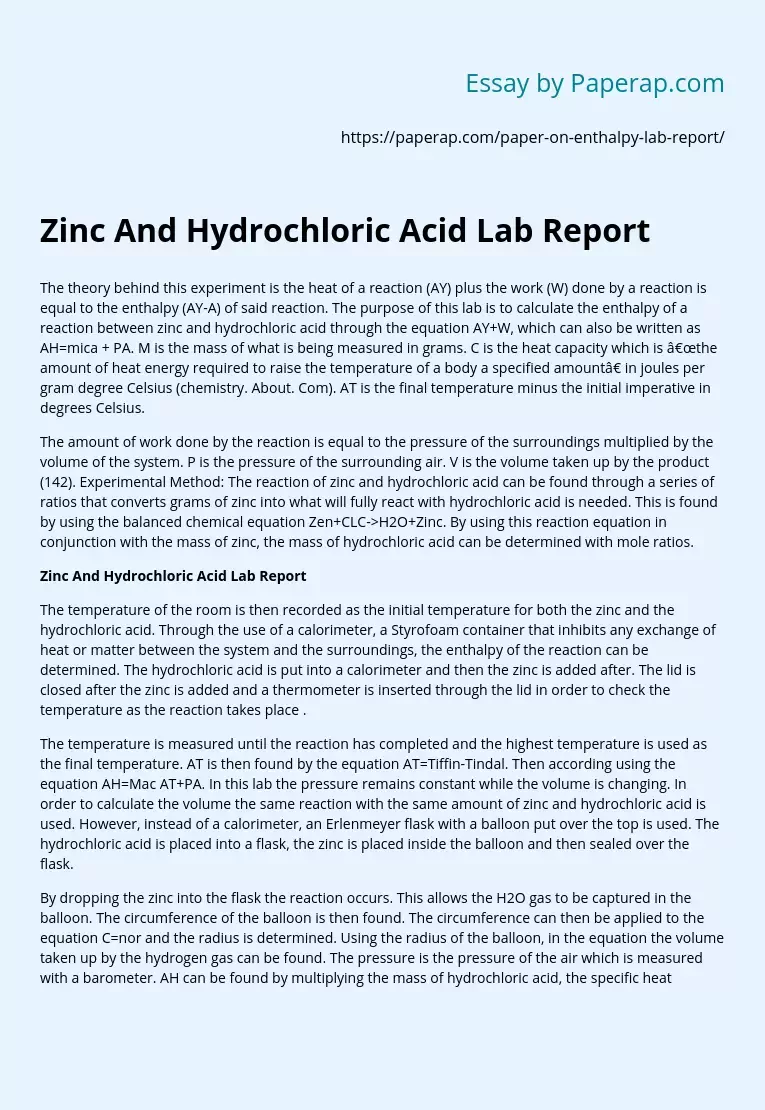# Zinc And Hydrochloric Acid Lab Report

The theory behind this experiment is the heat of a reaction (AY) plus the work (W) done by a reaction is equal to the enthalpy (AY-A) of said reaction. The purpose of this lab is to calculate the enthalpy of a reaction between zinc and hydrochloric acid through the equation AY+W, which can also be written as AH=mica + PA. M is the mass of what is being measured in grams. C is the heat capacity which is “the amount of heat energy required to raise the temperature of a body a specified amount” in joules per gram degree Celsius (chemistry.

About. Com). AT is the final temperature minus the initial imperative in degrees Celsius.

The amount of work done by the reaction is equal to the pressure of the surroundings multiplied by the volume of the system. P is the pressure of the surrounding air. V is the volume taken up by the product (142). Experimental Method: The reaction of zinc and hydrochloric acid can be found through a series of ratios that converts grams of zinc into what will fully react with hydrochloric acid is needed.

This is found by using the balanced chemical equation Zen+CLC->H2O+Zinc. By using this reaction equation in conjunction with the mass of zinc, the mass of hydrochloric acid can be determined with mole ratios.

## Zinc And Hydrochloric Acid Lab Report

The temperature of the room is then recorded as the initial temperature for both the zinc and the hydrochloric acid.

Get quality help nowDr. Karlyna PhDVerified

Proficient in: Chemical Reaction4.7 (235)

“ Amazing writer! I am really satisfied with her work. An excellent price as well. ”+84 relevant experts are online

Through the use of a calorimeter, a Styrofoam container that inhibits any exchange of heat or matter between the system and the surroundings, the enthalpy of the reaction can be determined. The hydrochloric acid is put into a calorimeter and then the zinc is added after. The lid is closed after the zinc is added and a thermometer is inserted through the lid in order to check the temperature as the reaction takes place .

The temperature is measured until the reaction has completed and the highest temperature is used as the final temperature. AT is then found by the equation AT=Tiffin-Tindal. Then according using the equation AH=Mac AT+PA. In this lab the pressure remains constant while the volume is changing. In order to calculate the volume the same reaction with the same amount of zinc and hydrochloric acid is used. However, instead of a calorimeter, an Erlenmeyer flask with a balloon put over the top is used. The hydrochloric acid is placed into a flask, the zinc is placed inside the balloon and then sealed over the flask.

By dropping the zinc into the flask the reaction occurs. This allows the H2O gas to be captured in the balloon. The circumference of the balloon is then found. The circumference can then be applied to the equation C=nor and the radius is determined. Using the radius of the balloon, in the equation the volume taken up by the hydrogen gas can be found. The pressure is the pressure of the air which is measured with a barometer. AH can be found by multiplying the mass of hydrochloric acid, the specific heat of HCI, and AT of the hydrochloric acid then dividing it by the moles of zinc.